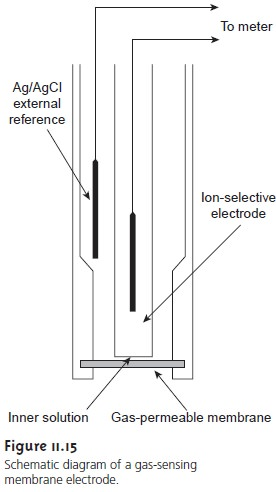Home | | Modern Analytical Chemistry | Gas-Sensing Electrodes - Potentiometric Methods of Analysis

# Gas-Sensing Electrodes - Potentiometric Methods of AnalysisA number of membrane electrodes have been devel- oped that respond to the concentration of dissolved gases.

Gas-Sensing Electrodes

A number of membrane electrodes have been devel- oped that respond to the concentration of dissolved gases. The basic design of these electrodes is shown in Figure 11.15 and consists of a thin membrane sepa- rating the sample from an inner solution containing an ion-selective electrode. The membrane is permeable to the gaseous analyte, but is not permeable to nonvolatile components in the sample matrix. Once the gaseous analyte passes through the membrane, it reacts in the inner solution, producing a species whose concentration can be monitored by an appropriate ion-selective elec- trode. For example, in the CO2 electrode, CO2 reacts in the inner solution to produce H3O+.3

CO2(aq)+ 2H2O(l) < = = = = > HCO3–(aq)+ H3O+(aq)

The change in the concentration of H3O+ is monitored with a pH ion-selective electrode, for which the cell potential is given by equation 11.9. The relation- ship between the concentration of H3O+ and CO2 is given by rearranging the equilibrium constant expression for reaction 11.10; thus11.11

where K is the equilibrium constant. If the amount of HCO3– in the internal solu

 3

tion is sufficiently large, then its concentration is unaffected by the presence of CO2 and remains constant. Substituting equation 11.11 into equation 11.9 gives

Ecell = K’ + 0.05916 log [CO2]

where K is a constant that includes the constant for the pH ion-selective electrode, the equilibrium constant for reaction 11.10, and the concentration of HCO3–.

Gas-sensing electrodes have been developed for a variety of gases, the charac- teristics for which are listed in Table 11.4. The composition of the inner solution changes with use, and both it and the membrane must be replaced periodically. Gas-sensing electrodes are stored in a solution similar to the internal solution to minimize their exposure to atmospheric gases.Study Material, Lecturing Notes, Assignment, Reference, Wiki description explanation, brief detail
Modern Analytical Chemistry: Electrochemical Methods of Analysis : Gas-Sensing Electrodes - Potentiometric Methods of Analysis |